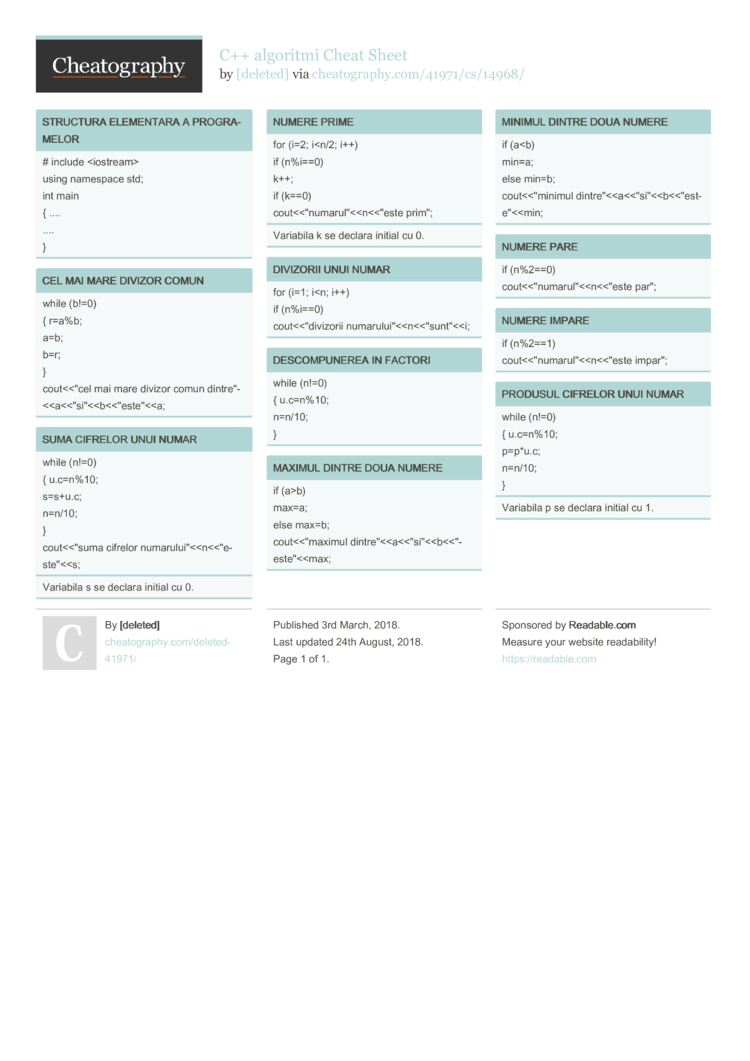# C++ algoritmi Cheat Sheet by [deleted]

### STRUCTURA ELEMENTARA A PROGRA­MELOR

 # include using namespace std; int main { .... .... }

### CEL MAI MARE DIVIZOR COMUN

 while (b!=0) { r=a%b; a=b; b=r; } cout<<­"cel mai mare divizor comun dintre­"­<

### SUMA CIFRELOR UNUI NUMAR

 while (n!=0) { u.c=n%10; s=s+u.c; n=n/10; } cout<<­"suma cifrelor numaru­lui­"­<
Variabila s se declara initial cu 0.

### NUMERE PRIME

 for (i=2; i
Variabila k se declara initial cu 0.

### DIVIZORII UNUI NUMAR

 for (i=1; i

### DESCOM­PUNEREA IN FACTORI

 while (n!=0) { u.c=n%10; n=n/10; }

### MAXIMUL DINTRE DOUA NUMERE

 if (a>b) max=a; else max=b; cout<<­"­maximul dintre­"­<

 if (a

### NUMERE PARE

 if (n%2==0) cout<<­"­num­aru­l"<<­n<<­"este par";

### NUMERE IMPARE

 if (n%2==1) cout<<­"­num­aru­l"<<­n<<­"este impar";

### PRODUSUL CIFRELOR UNUI NUMAR

 while (n!=0) { u.c=n%10; p=p*u.c; n=n/10; }
Variabila p se declara initial cu 1.1 Page
//media.cheatography.com/storage/thumb/deleted-41971_c-algoritmi.750.jpg

PDF (recommended)